866字，阅读需时3分钟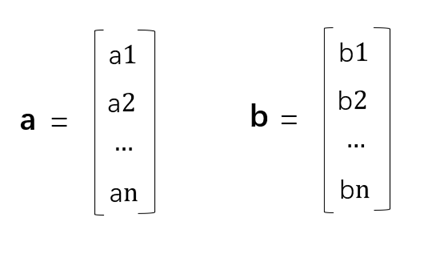a ● b = a1*b1 + a2 * b2 + …… + an * bn

e ●  f = 2 * 3 + (-1)*5 = 1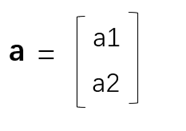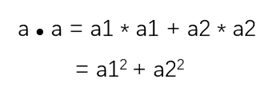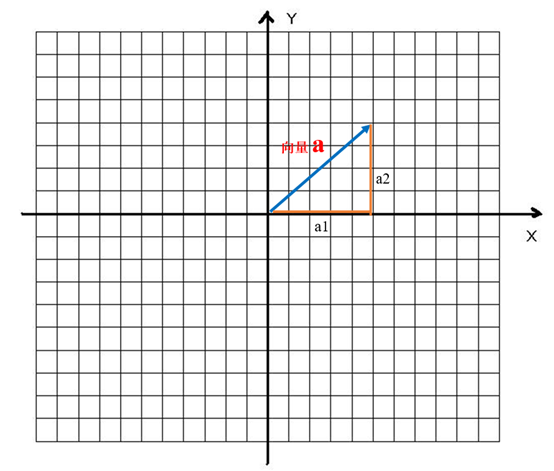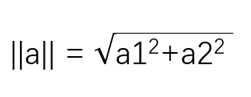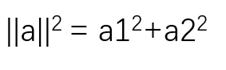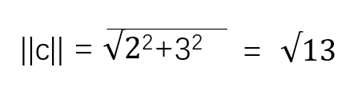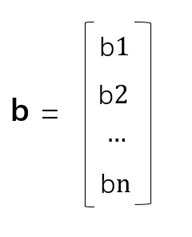n维向量b的模长为：

```import numpy as np
#创建向量a
a =  np.array([2,-1])
#创建向量b
b =  np.array([3,5])
if __name__ == '__main__':
#输出a与b的点积
print(np.dot(a,b))
#输出a的模长,对a自身的点积做开方运算
print(np.sqrt(np.dot(a,a)))```